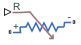# Variable Resistor

Linear variable resistor in electrical systems

•Libraries:
Simscape / Foundation Library / Electrical / Electrical Elements

## Description

The Variable Resistor block models a linear variable resistor, described with the following equation:

`$V=I·R$`

where:

• V is voltage.

• I is current.

• R is resistance, that is, the signal value at the control port.

Connections + and - are conserving electrical ports corresponding to the positive and negative terminals of the resistor, respectively. R is a physical signal input port that controls the resistance value. The current is positive if it flows from positive to negative, and the voltage across the resistor is equal to the difference between the voltage at the positive and the negative terminal, V(+) – V(–).

The optional thermal port H lets you connect the resistor to a thermal network. To expose the thermal port, select the Add thermal port check box and specify the thermal mass of the resistor by using the Thermal mass parameter. You can also specify the initial target for the Temperature variable. The block does not model other effects, such as the changes in resistance based on temperature.

### Variables

To set the priority and initial target values for the block variables prior to simulation, use the Initial Targets section in the block dialog box or Property Inspector. For more information, see Set Priority and Initial Target for Block Variables.

Nominal values provide a way to specify the expected magnitude of a variable in a model. Using system scaling based on nominal values increases the simulation robustness. Nominal values can come from different sources, one of which is the Nominal Values section in the block dialog box or Property Inspector. For more information, see Modify Nominal Values for a Block Variable.

## Ports

### Input

expand all

Input physical signal that specifies the resistance value.

### Conserving

expand all

Electrical conserving port associated with the resistor positive terminal.

Electrical conserving port associated with the resistor negative terminal.

Thermal conserving port that provides the resistor temperature.

#### Dependencies

To enable this port, select the Add thermal port check box.

## Parameters

expand all

The minimum resistance value. If the input signal falls below this level (for example, turns negative), this minimum resistance value is used. The parameter value must be nonnegative.

Selecting this check box exposes the thermal port H, which lets you connect the resistor to a thermal network. Selecting this check box also enables the Thermal mass parameter and adds the Temperature variable to the Initial Targets and Nominal Values sections.

Thermal mass of the resistor.

#### Dependencies

To enable this parameter, select the Add thermal port check box.

## Version History

Introduced in R2007a

expand all